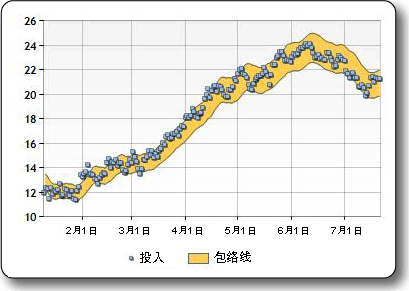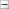• 包络线和包络谱计算程序
千次阅读
2020-12-04 11:52:35

%

一个求包络线和包络谱的程序

%

现代希尔伯特变换解调分析：

%

带通滤波；希尔伯特变换获得信号时域的包络线；用

fft

变换获得包络谱

%

如何获得包络线？

%

信号经希尔伯特变换不能直接得到包络，设信号

x

的希尔伯特变换为

y

，则平方和

%x.^2+y.^2(

或者再开根号，直接取平方和的效果为好

)

才是信号

x

的包络。

%

构造实验数据

clear all;close all;

t=0:0.005:1*pi;

fs=10000;

s=4*sin(2*200*pi*t).*(sin(2*4500*pi*t))+25*(sin(2*4500*pi*t));

figure(1);

subplot(211);plot(t,s);title('

原始信号

');

%

运用小波方法滤波

[c,l]=wavedec(s,1,'db10');

d1=wrcoef('d',c,l,'db10');

a1=0;

subplot(212);plot(d1);title('

滤波后重构的高频信号

');

%

希尔伯特变换求包络线

y=hilbert(d1);

y1=abs(d1+y*j);               %

这是取得包络线的三种方程。看一看哪种效果好。

%y1=abs(y);                     %

或者

z=x.^2+y.^2;

有的取得是

abs(y),

但是不推荐用。

%y1=d1.^2+y.^2;                 %

通过分析，

该方程在包络谱中的效果最好，

即取二者平

方和。

figure(2);

subplot(211);

hold on

plot(t,s);

plot(t,y1,'r');title('

包络线

');

hold off

%FFT

求包络谱

N=1024;

p=abs(fft(y1,N));

subplot(212);

plot((0:N/2-1)/N*fs,p(1:N/2));%

只需取到半频，即

fs/2

%f=(0:N-1)*fs/N; plot(f,p);   %

横坐标是在

fs

上，其中以

fs/2

为轴中心对称。

title('

包络谱

');xlabel('

频率

');ylabel('

功率谱

');

%

对比信号直接的傅里叶变换功率谱与包络谱

更多相关内容
• ## 包络线公式

千次阅读 2021-06-29 09:57:09
包络线公式08/04/2015本文内容包络线公式计算对一条移动平均线分别加上和减去一个指定百分比偏移而在其上方和下方绘出的“包络线”。包络线指标用于创建买卖信号。可以指定公式用于计算包络线的百分比。公式详细信息...

包络线公式

08/04/2015

本文内容

包络线公式计算对一条移动平均线分别加上和减去一个指定百分比偏移而在其上方和下方绘出的“包络线”。包络线指标用于创建买卖信号。可以指定公式用于计算包络线的百分比。公式详细信息语法

Chart.DataManipulator.FinancialFormula(

FinancialFormula.Envelopes,

"Period,Shift",

"Price",

"Upper:Lower")参数

此公式采用两个必需参数。

Period

用于计算移动平均线的时段。

Shift

用于从移动平均线加上或减去上方和下方包络线的百分比。输入值

此公式采用一个输入 Y 值。

Price

为其计算包络线的价格。输出值

此公式输出两个 Y 值。

Upper

上方包络线。

Lower

下方包络线。

备注

范围图是一种可方便地显示公式输出的图表类型。您可以使用折线图图表类型将上方包络线和下方包络线显示为两个数据序列。

示例

下面的示例采用 Series1 的第二个 Y 值输入 (Series1:Y2)，然后在 Series2 上输出 20 日移动平均线并在 Series3 上输出移动平均线的 7% 包络线。

' Calculate 20 days Simple moving average.

Chart1.DataManipulator.FinancialFormula (FinancialFormula.MovingAverage, "20", "Series1:Y2", "Series2:Y")

' Calculate 20 days Envelopes with 7 percent shift.

Chart1.DataManipulator.FinancialFormula (FinancialFormula.Envelopes, "20,7", "Series1:Y2", "Series3:Y,Series3:Y2")

// Calculate 20 days Simple moving average.

Chart1.DataManipulator.FinancialFormula (FinancialFormula.MovingAverage, "20", "Series1:Y2", "Series2:Y");

// Calculate 20 days Envelopes with 7 percent shift.

Chart1.DataManipulator.FinancialFormula (FinancialFormula.Envelopes, "20,7", "Series1:Y2", "Series3:Y,Series3:Y2");

请参见

参考

概念

展开全文• 信号 包络线 信号 MATLAB的 M 文件 验证过的很好用
• 包络线公式(图表控件)02/28/2011本文内容包络线公式计算对一条移动平均线分别加上和减去一个指定百分比偏移而在其上方和下方绘出的“包络线”。包络线指标用于创建买卖信号。可以指定公式用于计算包络线的百分比。...

包络线公式(图表控件)

02/28/2011

本文内容

包络线公式计算对一条移动平均线分别加上和减去一个指定百分比偏移而在其上方和下方绘出的“包络线”。包络线指标用于创建买卖信号。可以指定公式用于计算包络线的百分比。公式详细信息

语法

Chart.DataManipulator.FinancialFormula(

FinancialFormula.Envelopes,

"Period,Shift",

"Price",

"Upper:Lower")

参数

此公式采用两个必需参数。

Period

用于计算移动平均线的时段。

Shift

用于从移动平均线加上或减去上方和下方包络线的百分比。

输入值

此公式采用一个输入 Y 值。

Price

为其计算包络线的价格。

输出值

此公式输出两个 Y 值。

Upper

上方包络线。

Lower

下方包络线。

注释

范围图是一种可方便地显示公式输出的图表类型。您还可以使用折线图图表类型将上方包络线和下方包络线显示为两个数据序列。

示例

下面的示例采用 Series1 的第二个 Y 值输入 (Series1:Y2)，然后在 Series2 上输出 20 日移动平均线并在 Series3 上输出移动平均线的 7% 包络线。

' Calculate 20 days Simple moving average.

Chart1.DataManipulator.FinancialFormula (FinancialFormula.MovingAverage, "20", "Series1:Y2", "Series2:Y")

' Calculate 20 days Envelopes with 7 percent shift.

Chart1.DataManipulator.FinancialFormula (FinancialFormula.Envelopes, "20,7", "Series1:Y2", "Series3:Y,Series3:Y2")

// Calculate 20 days Simple moving average.

Chart1.DataManipulator.FinancialFormula (FinancialFormula.MovingAverage, "20", "Series1:Y2", "Series2:Y");

// Calculate 20 days Envelopes with 7 percent shift.

Chart1.DataManipulator.FinancialFormula (FinancialFormula.Envelopes, "20,7", "Series1:Y2", "Series3:Y,Series3:Y2");

请参阅

参考

其他资源

展开全文• u_x = [0,] #上包络的x序列 u_y = [s,] #上包络的y序列 l_x = [0,] #下包络的x序列 l_y = [s,] #下包络的y序列 # 检测波峰和波谷，并分别标记它们在u_x,u_y,l_x,l_中的位置。 #Detect peaks and troughs and ...

import math

import numpy as np

from numpy import array, sign, zeros

from scipy.interpolate import interp1d

import scipy.signal

#输入信号序列即可(list)

def envelope_extraction(signal):

s = signal.astype(float )

q_u = np.zeros(s.shape)

q_l = np.zeros(s.shape)

#在插值值前加上第一个值。这将强制模型对上包络和下包络模型使用相同的起点。

#Prepend the first value of (s) to the interpolating values. This forces the model to use the same starting point for both the upper and lower envelope models.

u_x = [0,] #上包络的x序列

u_y = [s,] #上包络的y序列

l_x = [0,] #下包络的x序列

l_y = [s,] #下包络的y序列

# 检测波峰和波谷，并分别标记它们在u_x,u_y,l_x,l_中的位置。

#Detect peaks and troughs and mark their location in u_x,u_y,l_x,l_y respectively.

for k in range(1,len(s)-1):

if (sign(s[k]-s[k-1])==1) and (sign(s[k]-s[k+1])==1):

u_x.append(k)

u_y.append(s[k])

if (sign(s[k]-s[k-1])==-1) and ((sign(s[k]-s[k+1]))==-1):

l_x.append(k)

l_y.append(s[k])

u_x.append(len(s)-1) #上包络与原始数据切点x

u_y.append(s[-1]) #对应的值

l_x.append(len(s)-1) #下包络与原始数据切点x

l_y.append(s[-1]) #对应的值

#u_x,l_y是不连续的，以下代码把包络转为和输入数据相同大小的数组[便于后续处理，如滤波]

upper_envelope_y = np.zeros(len(signal))

lower_envelope_y = np.zeros(len(signal))

upper_envelope_y = u_y#边界值处理

upper_envelope_y[-1] = u_y[-1]

lower_envelope_y = l_y#边界值处理

lower_envelope_y[-1] = l_y[-1]

#上包络

last_idx,next_idx = 0, 0

k, b = general_equation(u_x, u_y, u_x, u_y) #初始的k,b

for e in range(1, len(upper_envelope_y)-1):

if e not in u_x:

v = k * e + b

upper_envelope_y[e] = v

else:

idx = u_x.index(e)

upper_envelope_y[e] = u_y[idx]

last_idx = u_x.index(e)

next_idx = u_x.index(e) + 1

#求连续两个点之间的直线方程

k, b = general_equation(u_x[last_idx], u_y[last_idx], u_x[next_idx], u_y[next_idx])

#下包络

last_idx,next_idx = 0, 0

k, b = general_equation(l_x, l_y, l_x, l_y) #初始的k,b

for e in range(1, len(lower_envelope_y)-1):

if e not in l_x:

v = k * e + b

lower_envelope_y[e] = v

else:

idx = l_x.index(e)

lower_envelope_y[e] = l_y[idx]

last_idx = l_x.index(e)

next_idx = l_x.index(e) + 1

#求连续两个切点之间的直线方程

k, b = general_equation(l_x[last_idx], l_y[last_idx], l_x[next_idx], l_y[next_idx])

#也可以使用三次样条进行拟合

#u_p = interp1d(u_x,u_y, kind = 'cubic',bounds_error = False, fill_value=0.0)

#l_p = interp1d(l_x,l_y, kind = 'cubic',bounds_error = False, fill_value=0.0)

#for k in range(0,len(s)):

# q_u[k] = u_p(k)

# q_l[k] = l_p(k)

return upper_envelope_y, lower_envelope_y

def general_equation(first_x,first_y,second_x,second_y):

# 斜截式 y = kx + b

A = second_y-first_y

B = first_x-second_x

C = second_x * first_y - first_x * second_y

k = -1 * A / B

b = -1 * C / B

return k, b

展开全文• 使用逐次逼近法计算瞬态恢复电压波形的包络线.pdf云计算
• 全自动驾驶车辆动态包络线理论计算与试验研究.pdf
• ## MATLAB - 数字信号包络线的求取

千次阅读 多人点赞 2021-01-20 16:59:24
函数： %%%%%%%%%%%%%%%%%%%%%%%%%%%%%%%%%%%%%%%%%%%%%%%%%%...% 这个函数首先移除x的均值，然后在计算包络线之后再把它加回来。 % % 如果x是一个矩阵，那么包络线对x的每一列都是独立的。 % %%%%%%%%%%%%%%%%%%%%%%%%信号 matlab
• 在此背景下，提出了扩展的包络条件来适应C0连续的曲线包络计算，并证明了在C1连续条件下扩展包络条件是传统包络条件的充分非必要条件；在此基础上，提出了一种新的任意非凸平面多边形变形包络计算方法。最后，...
• Python-绘制曲线的包络线 之前分享过matlab如何绘制包络线（传送门：Matlab绘制信号包络线），今天分享一下python如何实现 包络线基于scipy库，利用scipy.signal.hilbert 用法： scipy.signal.hilbert（x，N=...matlab python
• ## matlab包络线函数

千次阅读 2021-04-18 10:01:39
过大 脑 的取舍 ,...(c )可 以看 出,经过 MATLAB 中的 Hilbert 函数 后也 出现 了皱 纹 ,......ymax])函数来调整图轴的范围 axis([0,6,-1.5,1]) 6 MATLAB 也可对图形...(-x)') 9 画出衰减振荡曲线与它的包络线及。...
• 针对当前车辆动态包络线的获取主要通过计算手段这一情况，设计了高速列车动态包络线测量系统。系统基于双目视觉测量原理，利用大功率线激光瞬时光源构造测量特征，通过高速采集、处理、解算能反映列车动态偏移的被测...
• 70% 乙烷和 30% 苯）的两种二元混合物的相包络。 这个二元系统表现出一种称为逆行冷凝的特殊行为（例如，当我们升高温度或降低压力时会发生冷凝）。 我们使用 SRK 状态方程来计算平衡常数。 临界点是从 HYSYS 3.2 中...matlab
• ## python 插值求包络线

千次阅读 2022-03-21 22:56:55
SciPy 是 Python 里处理科学计算 (scientific computing) 的包，使用它遇到问题可访问它的官网 (https://www.scipy.org/). 去找答案。 在使用 scipy 之前，需要引进它，语法如下： import ...python
• 在深入研究和分析EMD算法的基础上,提出了一种基于波形匹配的端点效应处理方案,通过计算波形匹配度, 在平均包络线内部寻找与其端部变化趋势最为接近的子波,并用这段子波代替平均包络线的边缘部分, 使处理后的平均...
• 为了更加便捷地获取车辆的动态包络线,研究出一种基于Matlab软件与三维空间几何变换...该研究方法,使得车辆动态包络线的获取更便捷,最终结果更切合实际,从而更加充分地利用限界的有效空间,也为车辆限界计算提供新思路。
• 使用ATR的趋势包络线指标计算了价格的变化，在计算之前加上对价格的平滑处理。
• 自动包络通过计算最后 n 根柱线的标准偏差来自动调整通道大小。
• 光谱去除包络线的目的：在光谱曲线相似的情况下，直接从中提取光谱数据特征不便于计算，因此需要对光谱曲线做进一步处理，以突出光谱特征。包络线消除法可以有效突出光谱曲线的吸收、反射和发射特征，并将其归一到一...算法 matlab
• 信号包络熵的仿真计算及特点分析，计算信号的包络谱熵值，提取最小包络
• %% 数据边界 clear all; close all;clc; % %% 数据 data = load ('ftp_75_gear.txt'); %导入工况数据 data=data(1:end,:); time=data(:,1);...%% 求出曲线的垂直距离为2的包络线 x=time; y=speed; r=2; r...
• 若不用会怎样 plot(x,y),xlabel('x'),ylabel('y'),title('y=x*exp(-x)') 9 画出衰减振荡曲线与它的包络线及。t 的取值范围是[0, 4π] 。 t=......【总页数】3 页(55-57) 【关键词】渐开线;范成法;MATLAB;包络线 ...
•  （2）以最大值点作为包络线的一个端点，计算该点与长波方向（波长增长的方向）各个极大值连线的斜率，以斜率最大点作为包络线下一个端点，再以此点为起点循环，直至最后一点； （3）以最大值点作为包选线的一个...遥感图像 c++
• ） 资料包络分析法 PPT 资料包络分析法 更多扩展方法介绍。 另外，从事效率生产率研究与学习的入门教材是Timothy J. Coelli.的 Introduction to Efficiency and Productivity Analysis (2nd edition) 也可以借鉴学习...
• 计算当前点与左边所有点连线的斜率取斜率最小的连线的端点作为包络线的下一个端点，同时更新当前点为该端点，重复此过程，直到第一个点。 4.直到所有极大值点都加入包络线为止 MATLAB代码 main.m %Copyright (C) ...算法
• 狭义的检波是指从调幅波的包络提取调制信号的过程，这种检波方法也被称为包络检波。希尔伯特变换可以用作包络检波。希尔伯特变换Hilbert 变换能在振幅保持不变的情况下将输入信号的相角偏移 90 度，简单地说就是能够...
• clear all; clc; close all; fs=400; % 采样频率 N=400; % 数据长度 n=0:1:N-1; dt=1/fs; t=n*dt; % 时间序列 A=0.5; % 相位调制幅值 x=(1+... % 包络线 figure; plot(x);hold on ;plot(a); hold on; plot((-1)*a,'b')
• 好多地方都有求数据包络线的程序这里不再赘述，比如链接： 添加链接描述 但是对于时间序列数据我们求包络线的过程中有时候想保留对应的时间值。 上面链接中第三步： 第一个问题： “包络线的生成，需要将原数据分成...matlab 算法...